# Calculus for Electric Circuits

## Mathematics for Electronics

• #### Question 1

Calculus is a branch of mathematics that originated with scientific questions concerning rates of change. The easiest rates of change for most people to understand are those dealing with time. For example, a student watching their savings account dwindle over time as they pay for tuition and other expenses is very concerned with rates of change (dollars per year being spent).

In calculus, we have a special word to describe rates of change: derivative. One of the notations used to express a derivative (rate of change) appears as a fraction. For example, if the variable S represents the amount of money in the student’s savings account and t represents time, the rate of change of dollars over time would be written like this:

 dS dt

The following set of figures puts actual numbers to this hypothetical scenario:

Date: November 20
Saving account balance (S) = \$12,527.33
Rate of spending ([dS/dt]) = -5,749.01 per year

List some of the equations you have seen in your study of electronics containing derivatives, and explain how rate of change relates to the real-life phenomena described by those equations.

• #### Question 2

According to the “Ohm’s Law” formula for a capacitor, capacitor current is proportional to the time-derivative of capacitor voltage:

 i = C dv dt

Another way of saying this is to state that the capacitors differentiate voltage with respect to time, and express this time-derivative of voltage as a current.

Suppose we had an oscilloscope capable of directly measuring current, or at least a current-to-voltage converter that we could attach to one of the probe inputs to allow direct measurement of current on one channel. With such an instrument set-up, we could directly plot capacitor voltage and capacitor current together on the same display: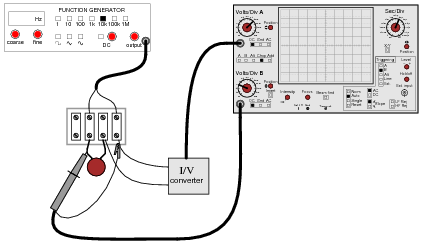For each of the following voltage waveforms (channel B), plot the corresponding capacitor current waveform (channel A) as it would appear on the oscilloscope screen: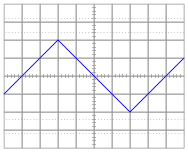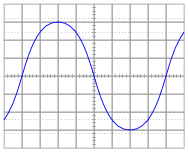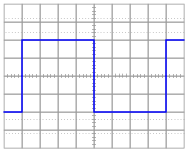Note: the amplitude of your current plots is arbitrary. What I’m interested in here is the shape of each current waveform!

• #### Question 3

Potentiometers are very useful devices in the field of robotics, because they allow us to represent the position of a machine part in terms of a voltage. In this particular case, a potentiometer mechanically linked to the joint of a robotic arm represents that arm’s angular position by outputting a corresponding voltage signal: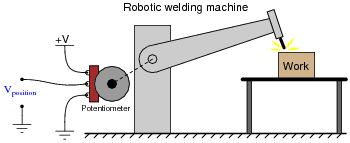As the robotic arm rotates up and down, the potentiometer wire moves along the resistive strip inside, producing a voltage directly proportional to the arm’s position. A voltmeter connected between the potentiometer wiper and ground will then indicate arm position. A computer with an analog input port connected to the same points will be able to measure, record, and (if also connected to the arm’s motor drive circuits) control the arm’s position.

If we connect the potentiometer’s output to a differentiator circuit, we will obtain another signal representing something else about the robotic arm’s action. What physical variable does the differentiator output signal represent?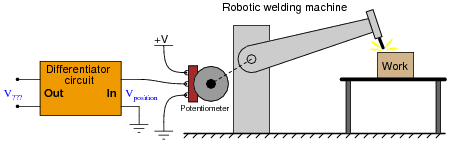• #### Question 4

One of the fundamental principles of calculus is a process called integration. This principle is important to understand because it is manifested in the behavior of capacitance. Thankfully, there are more familiar physical systems which also manifest the process of integration, making it easier to comprehend.

If we introduce a constant flow of water into a cylindrical tank with water, the water level inside that tank will rise at a constant rate over time: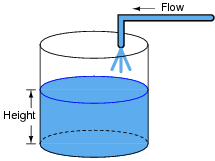In calculus terms, we would say that the tank integrates water flow into water height. That is, one quantity (flow) dictates the rate-of-change over time of another quantity (height).

Like the water tank, electrical capacitance also exhibits the phenomenon of integration with respect to time. Which electrical quantity (voltage or current) dictates the rate-of-change over time of which other quantity (voltage or current) in a capacitance? Or, to re-phrase the question, which quantity (voltage or current), when maintained at a constant value, results in which other quantity (current or voltage) steadily ramping either up or down over time?

• #### Question 5

One of the fundamental principles of calculus is a process called integration. This principle is important to understand because it is manifested in the behavior of inductance. Thankfully, there are more familiar physical systems which also manifest the process of integration, making it easier to comprehend.

If we introduce a constant flow of water into a cylindrical tank with water, the water level inside that tank will rise at a constant rate over time:In calculus terms, we would say that the tank integrates water flow into water height. That is, one quantity (flow) dictates the rate-of-change over time of another quantity (height).

Like the water tank, electrical inductance also exhibits the phenomenon of integration with respect to time. Which electrical quantity (voltage or current) dictates the rate-of-change over time of which other quantity (voltage or current) in an inductance? Or, to re-phrase the question, which quantity (voltage or current), when maintained at a constant value, results in which other quantity (current or voltage) steadily ramping either up or down over time?

• #### Question 6

Both the input and the output of this circuit are square waves, although the output waveform is slightly distorted and also has much less amplitude: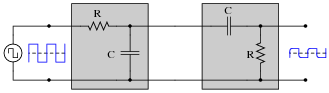You recognize one of the RC networks as a passive integrator, and the other as a passive differentiator. What does the likeness of the output waveform compared to the input waveform indicate to you about differentiation and integration as functions applied to waveforms?

• #### Question 7

Determine what the response will be to a constant DC voltage applied at the input of these (ideal) circuits: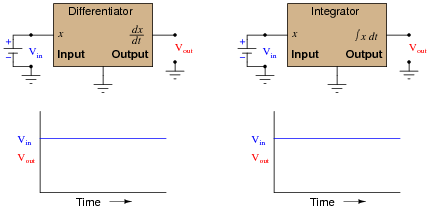• #### Question 8

In calculus, differentiation is the inverse operation of something else called integration. That is to say, differentiation “un-does” integration to arrive back at the original function (or signal). To illustrate this electronically, we may connect a differentiator circuit to the output of an integrator circuit and (ideally) get the exact same signal out that we put in: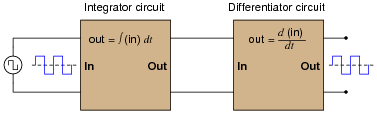Based on what you know about differentiation and differentiator circuits, what must the signal look like in between the integrator and differentiator circuits to produce a final square-wave output? In other words, if we were to connect an oscilloscope in between these two circuits, what sort of signal would it show us?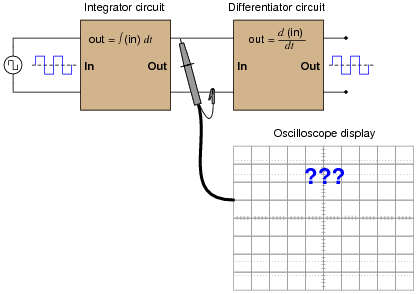• #### Question 9

Plot the relationships between voltage and current for resistors of three different values (1 Ω, 2 Ω, and 3 Ω), all on the same graph: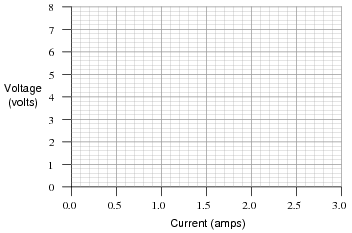What pattern do you see represented by your three plots? What relationship is there between the amount of resistance and the nature of the voltage/current function as it appears on the graph?

Advanced question: in calculus, the instantaneous rate-of-change of an (x,y) function is expressed through the use of the derivative notation: [dy/dx]. How would the derivative for each of these three plots be properly expressed using calculus notation? Explain how the derivatives of these functions relate to real electrical quantities.

• #### Question 10

Ohm’s Law tells us that the amount of current through a fixed resistance may be calculated as such:

 I = E R

We could also express this relationship in terms of conductance rather than resistance, knowing that G = 1/R:

 I = EG

However, the relationship between current and voltage for a fixed capacitance is quite different. The “Ohm’s Law” formula for a capacitor is as such:

 i = C de dt

What significance is there in the use of lower-case variables for current (i) and voltage (e)? Also, what does the expression [de/dt] mean? Note: in case you think that the d’s are variables, and should cancel out in this fraction, think again: this is no ordinary quotient! The d letters represent a calculus concept known as a differential, and a quotient of two d terms is called a derivative.

• #### Question 11

Capacitors store energy in the form of an electric field. We may calculate the energy stored in a capacitance by integrating the product of capacitor voltage and capacitor current (P = IV) over time, since we know that power is the rate at which work (W) is done, and the amount of work done to a capacitor taking it from zero voltage to some non-zero amount of voltage constitutes energy stored (U):

 P = dW dt

 dW = P dt

 U = W = ⌠ ⌡ P dt

Find a way to substitute capacitance (C) and voltage (V) into the integrand so you may integrate to find an equation describing the amount of energy stored in a capacitor for any given capacitance and voltage values.

• #### Question 12

Integrator circuits may be understood in terms of their response to DC input signals: if an integrator receives a steady, unchanging DC input voltage signal, it will output a voltage that changes with a steady rate over time. The rate of the changing output voltage is directly proportional to the magnitude of the input voltage: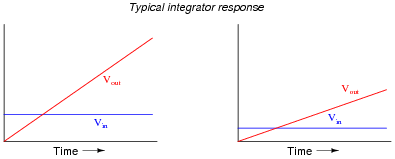A symbolic way of expressing this input/output relationship is by using the concept of the derivative in calculus (a rate of change of one variable compared to another). For an integrator circuit, the rate of output voltage change over time is proportional to the input voltage:

 dVout dt ∝ Vin

A more sophisticated way of saying this is, “The time-derivative of output voltage is proportional to the input voltage in an integrator circuit.” However, in calculus there is a special symbol used to express this same relationship in reverse terms: expressing the output voltage as a function of the input. For an integrator circuit, this special symbol is called the integration symbol, and it looks like an elongated letter “S”:

 Vout ∝ ⌠ ⌡ T 0 Vin dt

Here, we would say that output voltage is proportional to the time-integral of the input voltage, accumulated over a period of time from time=0 to some point in time we call T.

“This is all very interesting,” you say, “but what does this have to do with anything in real life?” Well, there are actually a great deal of applications where physical quantities are related to each other by time-derivatives and time-integrals. Take this water tank, for example:One of these variables (either height H or flow F, I’m not saying yet!) is the time-integral of the other, just as Vout is the time-integral of Vin in an integrator circuit. What this means is that we could electrically measure one of these two variables in the water tank system (either height or flow) so that it becomes represented as a voltage, then send that voltage signal to an integrator and have the output of the integrator derive the other variable in the system without having to measure it!

Your task is to determine which variable in the water tank scenario would have to be measured so we could electronically predict the other variable using an integrator circuit.

• #### Question 13

We know that the output of an integrator circuit is proportional to the time-integral of the input voltage:Vout ∝ ⌠ ⌡ T 0 Vin dt

But how do we turn this proportionality into an exact equality, so that it accounts for the values of R and C? Although the answer to this question is easy enough to simply look up in an electronics reference book, it would be great to actually derive the exact equation from your knowledge of electronic component behaviors! Here are a couple of hints:

 I = V R i = C dv dt

• #### Question 14

If an object moves in a straight line, such as an automobile traveling down a straight road, there are three common measurements we may apply to it: position (x), velocity (v), and acceleration (a). Position, of course, is nothing more than a measure of how far the object has traveled from its starting point. Velocity is a measure of how fast its position is changing over time. Acceleration is a measure of how fast the velocity is changing over time.

These three measurements are excellent illustrations of calculus in action. Whenever we speak of “rates of change,” we are really referring to what mathematicians call derivatives. Thus, when we say that velocity (v) is a measure of how fast the object’s position (x) is changing over time, what we are really saying is that velocity is the “time-derivative” of position. Symbolically, we would express this using the following notation:

 v = dx dt

Likewise, if acceleration (a) is a measure of how fast the object’s velocity (v) is changing over time, we could use the same notation and say that acceleration is the time-derivative of velocity:

 a = dv dt

Since it took two differentiations to get from position to acceleration, we could also say that acceleration is the second time-derivative of position:

 a = d2x dt2

“What has this got to do with electronics,” you ask? Quite a bit! Suppose we were to measure the velocity of an automobile using a tachogenerator sensor connected to one of the wheels: the faster the wheel turns, the more DC voltage is output by the generator, so that voltage becomes a direct representation of velocity. Now we send this voltage signal to the input of a differentiator circuit, which performs the time-differentiation function on that signal. What would the output of this differentiator circuit then represent with respect to the automobile, position or acceleration? What practical use do you see for such a circuit?

Now suppose we send the same tachogenerator voltage signal (representing the automobile’s velocity) to the input of an integrator circuit, which performs the time-integration function on that signal (which is the mathematical inverse of differentiation, just as multiplication is the mathematical inverse of division). What would the output of this integrator then represent with respect to the automobile, position or acceleration? What practical use do you see for such a circuit?

• #### Question 15

A familiar context in which to apply and understand basic principles of calculus is the motion of an object, in terms of position (x), velocity (v), and acceleration (a). We know that velocity is the time-derivative of position (v = [dx/dt]) and that acceleration is the time-derivative of velocity (a = [dv/dt]). Another way of saying this is that velocity is the rate of position change over time, and that acceleration is the rate of velocity change over time.

It is easy to construct circuits which input a voltage signal and output either the time-derivative or the time-integral (the opposite of the derivative) of that input signal. We call these circuits “differentiators” and “integrators,” respectively.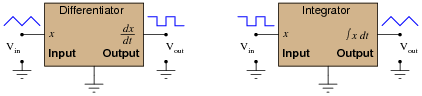Integrator and differentiator circuits are highly useful for motion signal processing, because they allow us to take voltage signals from motion sensors and convert them into signals representing other motion variables. For each of the following cases, determine whether we would need to use an integrator circuit or a differentiator circuit to convert the first type of motion signal into the second:

Converting velocity signal to position signal: (integrator or differentiator?)
Converting acceleration signal to velocity signal: (integrator or differentiator?)
Converting position signal to velocity signal: (integrator or differentiator?)
Converting velocity signal to acceleration signal: (integrator or differentiator?)
Converting acceleration signal to position signal: (integrator or differentiator?)

Also, draw the schematic diagrams for these two different circuits.

• #### Question 16

We know that the output of a differentiator circuit is proportional to the time-derivative of the input voltage:Vout ∝ dVin dt

But how do we turn this proportionality into an exact equality, so that it accounts for the values of R and C? Although the answer to this question is easy enough to simply look up in an electronics reference book, it would be great to actually derive the exact equation from your knowledge of electronic component behaviors! Here are a couple of hints:

 I = V R i = C dv dt

• #### Question 17

You are part of a team building a rocket to carry research instruments into the high atmosphere. One of the variables needed by the on-board flight-control computer is velocity, so it can throttle engine power and achieve maximum fuel efficiency. The problem is, none of the electronic sensors on board the rocket has the ability to directly measure velocity. What is available is an altimeter, which infers the rocket’s altitude (it position away from ground) by measuring ambient air pressure; and also an accelerometer, which infers acceleration (rate-of-change of velocity) by measuring the inertial force exerted by a small mass.

The lack of a “speedometer” for the rocket may have been an engineering design oversight, but it is still your responsibility as a development technician to figure out a workable solution to the dilemma. How do you propose we obtain the electronic velocity measurement the rocket’s flight-control computer needs?

• #### Question 18

A Rogowski Coil is essentially an air-core current transformer that may be used to measure DC currents as well as AC currents. Like all current transformers, it measures the current going through whatever conductor(s) it encircles.

Normally transformers are considered AC-only devices, because electromagnetic induction requires a changing magnetic field ([(d φ)/dt]) to induce voltage in a conductor. The same is true for a Rogowski coil: it produces a voltage only when there is a change in the measured current. However, we may measure any current (DC or AC) using a Rogowski coil if its output signal feeds into an integrator circuit as shown: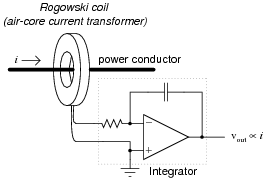Connected as such, the output of the integrator circuit will be a direct representation of the amount of current going through the wire.

Explain why an integrator circuit is necessary to condition the Rogowski coil’s output so that output voltage truly represents conductor current.

• #### Question 19

A Rogowski coil has a mutual inductance rating of 5 μH. Calculate the size of the resistor necessary in the integrator circuit to give the integrator output a 1:1 scaling with the measured current, given a capacitor size of 4.7 nF: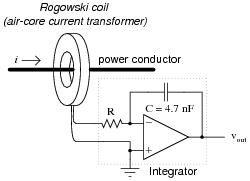That is, size the resistor such that a current through the conductor changing at a rate of 1 amp per second will generate an integrator output voltage changing at a rate of 1 volt per second.

• #### Question 20

The chain rule of calculus states that:

 dx dy dy dz = dx dz

Similarly, the following mathematical principle is also true:

dx

dy
=  dx dz

 dy dz

It is very easy to build an opamp circuit that differentiates a voltage signal with respect to time, such that an input of x produces an output of [dx/dt], but there is no simple circuit that will output the differential of one input signal with respect to a second input signal.

However, this does not mean that the task is impossible. Draw a block diagram for a circuit that calculates [dy/dx], given the input voltages x and y. Hint: this circuit will make use of differentiators.

Challenge question: draw a full opamp circuit to perform this function!

• #### Question 21

Ohm’s Law tells us that the amount of voltage dropped by a fixed resistance may be calculated as such:

 E = IR

However, the relationship between voltage and current for a fixed inductance is quite different. The “Ohm’s Law” formula for an inductor is as such:

 e = L di dt

What significance is there in the use of lower-case variables for current (i) and voltage (e)? Also, what does the expression [di/dt] mean? Note: in case you think that the d’s are variables, and should cancel out in this fraction, think again: this is no ordinary quotient! The d letters represent a calculus concept known as a differential, and a quotient of two d terms is called a derivative.

• #### Question 22

Digital logic circuits, which comprise the inner workings of computers, are essentially nothing more than arrays of switches made from semiconductor components called transistors. As switches, these circuits have but two states: on and off, which represent the binary states of 1 and 0, respectively.

The faster these switch circuits are able to change state, the faster the computer can perform arithmetic and do all the other tasks computers do. To this end, computer engineers keep pushing the limits of transistor circuit design to achieve faster and faster switching rates.

This race for speed causes problems for the power supply circuitry of computers, though, because of the current “surges” (technically called transients) created in the conductors carrying power from the supply to the logic circuits. The faster these logic circuits change state, the greater the [di/dt] rates-of-change exist in the conductors carrying current to power them. Significant voltage drops can occur along the length of these conductors due to their parasitic inductance:Suppose a logic gate circuit creates transient currents of 175 amps per nanosecond (175 A/ns) when switching from the “off” state to the “on” state. If the total inductance of the power supply conductors is 10 picohenrys (9.5 pH), and the power supply voltage is 5 volts DC, how much voltage remains at the power terminals of the logic gate during one of these “surges”?

• #### Question 23

Inductors store energy in the form of a magnetic field. We may calculate the energy stored in an inductance by integrating the product of inductor voltage and inductor current (P = IV) over time, since we know that power is the rate at which work (W) is done, and the amount of work done to an inductor taking it from zero current to some non-zero amount of current constitutes energy stored (U):

 P = dW dt

 dW = P dt

 U = W = ⌠ ⌡ P dt

Find a way to substitute inductance (L) and current (I) into the integrand so you may integrate to find an equation describing the amount of energy stored in an inductor for any given inductance and current values.

• #### Question 24

Define what “derivative” means when applied to the graph of a function. For instance, examine this graph: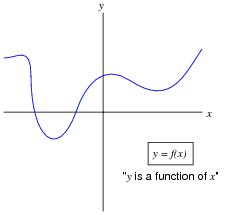Label all the points where the derivative of the function ([dy/dx]) is positive, where it is negative, and where it is equal to zero.

• #### Question 25

Shown here is the graph for the function y = x2: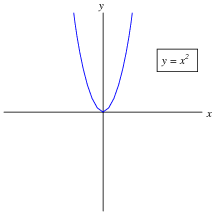Sketch an approximate plot for the derivative of this function.

• #### Question 26

Calculus is widely (and falsely!) believed to be too complicated for the average person to understand. Yet, anyone who has ever driven a car has an intuitive grasp of calculus’ most basic concepts: differentiation and integration. These two complementary operations may be seen at work on the instrument panel of every automobile: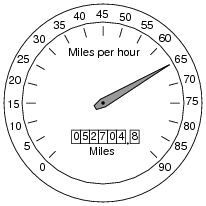On this one instrument, two measurements are given: speed in miles per hour, and distance traveled in miles. In areas where metric units are used, the units would be kilometers per hour and kilometers, respectively. Regardless of units, the two variables of speed and distance are related to each other over time by the calculus operations of integration and differentiation. My question for you is which operation goes which way?

We know that speed is the rate of change of distance over time. This much is apparent simply by examining the units (miles per hour indicates a rate of change over time). Of these two variables, speed and distance, which is the derivative of the other, and which is the integral of the other? Also, determine what happens to the value of each one as the other maintains a constant (non-zero) value.

• #### Question 27

Define what “integral” means when applied to the graph of a function. For instance, examine this graph: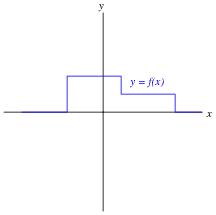Sketch an approximate plot for the integral of this function.

• #### Question 28

A forward-biased PN semiconductor junction does not possess a “resistance” in the same manner as a resistor or a length of wire. Any attempt at applying Ohm’s Law to a diode, then, is doomed from the start.

This is not to say that we cannot assign a dynamic value of resistance to a PN junction, though. The fundamental definition of resistance comes from Ohm’s Law, and it is expressed in derivative form as such:

 R = dV dI

The fundamental equation relating current and voltage together for a PN junction is Shockley’s diode equation:

 I = IS (e[qV/NkT] − 1)

At room temperature (approximately 21 degrees C, or 294 degrees K), the thermal voltage of a PN junction is about 25 millivolts. Substituting 1 for the non-ideality coefficient, we may simply the diode equation as such:

 I = IS (e[V/0.025] − 1) or I = IS (e40 V − 1)

Differentiate this equation with respect to V, so as to determine [dI/dV], and then reciprocate to find a mathematical definition for dynamic resistance ([dV/dI]) of a PN junction. Hints: saturation current (IS) is a very small constant for most diodes, and the final equation should express dynamic resistance in terms of thermal voltage (25 mV) and diode current (I).

• #### Question 29

Just as addition is the inverse operation of subtraction, and multiplication is the inverse operation of division, a calculus concept known as integration is the inverse function of differentiation. Symbolically, integration is represented by a long “S”-shaped symbol called the integrand:

 If x = dy dt ( x is the derivative of y with respect to t)

 Then y = ⌠ ⌡ x dt ( y is the integral of x with respect to t)

To be truthful, there is a bit more to this reciprocal relationship than what is shown above, but the basic idea you need to grasp is that integration “un-does” differentiation, and visa-versa. Derivatives are a bit easier for most people to understand, so these are generally presented before integrals in calculus courses. One common application of derivatives is in the relationship between position, velocity, and acceleration of a moving object. Velocity is nothing more than rate-of-change of position over time, and acceleration is nothing more than rate-of-change of velocity over time:

 v = dx dt Velocity (v ) is the time−derivative of position (x )

 a = dv dt Acceleration (a ) is the time−derivative of velocity (v )

Illustrating this in such a way that shows differentiation as a process: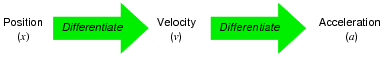Given that you know integration is the inverse-function of differentiation, show how position, velocity, and acceleration are related by integration. Show this both in symbolic (proper mathematical) form as well as in an illustration similar to that shown above.

### Footnotes:

It is perfectly accurate to say that differentiation undoes integration, so that [d/dt] ∫x dt = x, but to say that integration undoes differentiation is not entirely true because indefinite integration always leaves a constant C that may very well be non-zero, so that ∫[dx/dt] dt = x C rather than simply being x.

• #### Question 30

Calculus is a branch of mathematics that originated with scientific questions concerning rates of change. The easiest rates of change for most people to understand are those dealing with time. For example, a student watching their savings account dwindle over time as they pay for tuition and other expenses is very concerned with rates of change (dollars per day being spent).

The “derivative” is how rates of change are symbolically expressed in mathematical equations. For example, if the variable S represents the amount of money in the student’s savings account and t represents time, the rate of change of dollars over time (the time-derivative of the student’s account balance) would be written as [dS/dt]. The process of calculating this rate of change from a record of the account balance over time, or from an equation describing the balance over time, is called differentiation.

Suppose, though, that instead of the bank providing the student with a statement every month showing the account balance on different dates, the bank were to provide the student with a statement every month showing the rates of change of the balance over time, in dollars per day, calculated at the end of each day: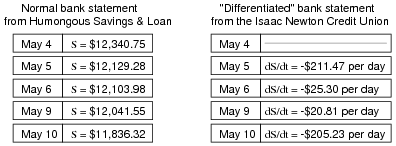Explain how the Isaac Newton Credit Union calculates the derivative ([dS/dt]) from the regular account balance numbers (S in the Humongous Savings & Loan statement), and then explain how the student who banks at Isaac Newton Credit Union could figure out how much money is in their account at any given time.

Hint: the process of calculating a variable’s value from rates of change is called integration in calculus. It is the opposite (inverse) function of differentiation.